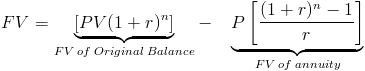# Remaining Balance on LoanThe formula for the remaining balance on a loan can be used to calculate the remaining balance at a given time(time n), whether at a future date or at present. The remaining balance on a loan formula shown is only used for a loan that is amortized, meaning that the portion of interest and principal applied to each payment is predetermined.

The term "future value" in the remaining balance formula may seem confusing, but the balance at any time after payments are being made is the future value in respect to the origination of the loan.

It is important, as with all financial formulas, that the interest rate per period and term relate to one another and to when the payments are made. With monthly payments, the rate would need to be the monthly rate and not the annual rate.

## Use of Remaining Balance Formula

Using this formula, a simple interest loan will return an incorrect answer in most cases because the portion of principal and interest is determined by the date the payment was made on.

It is also important to note that the remaining balance on a loan formula shown is only used for conventional loans where the payment, rate, and term are fixed. Specialized loans like graduating payment, option, and negative amortization will require special calculations to determine the loan balance at a particular time.

The remaining balance on a loan formula can be used with mortgages, consumer loans, and commercial loans. Where the money is spent does not have an affect on how to calculate the remaining balance on a loan.

## How is the Remaining Balance Formula derived?

The remaining balance of a loan formula can be separated into two sections, the future value of the original loan amount and the future value of the annuity.The future value of the original loan amount in the first section of the formula will determine what the value would be at time n if no payments were made.

A loan is an annuity, in that it is a series of periodic payments. Given this, the second section of the formula would be the future value of the payments made up until time n.

The remaining balance can then be calculated by subtracting the future value of the payments made from the future value of the original balance at time n.

New to Finance?